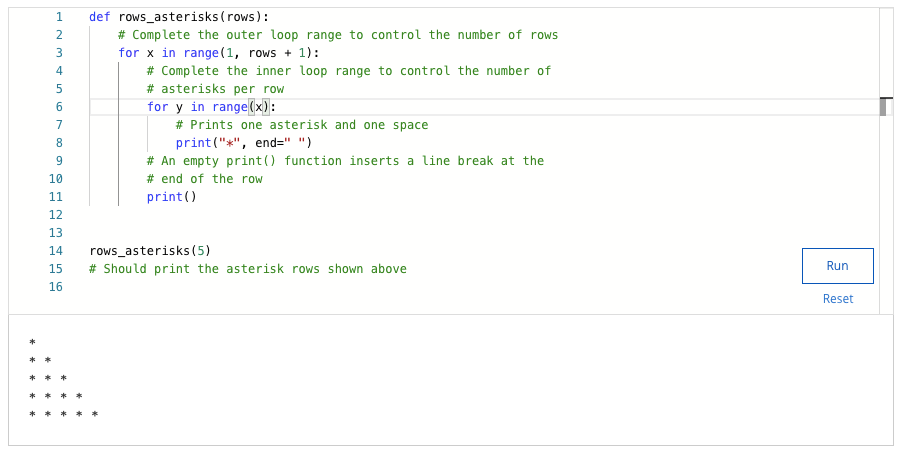## crash course on python week 3 quiz answers – Shuffle Q/A 1

#### 28. Fill in the blanks to complete the “even_numbers” function. This function should return a space-separated string of all positive even numbers, excluding 0, up to and including the “maximum” variable that's passed into the function. Complete the for loop so that a function call like “even_numbers(6)” will return the numbers “2 4 6”.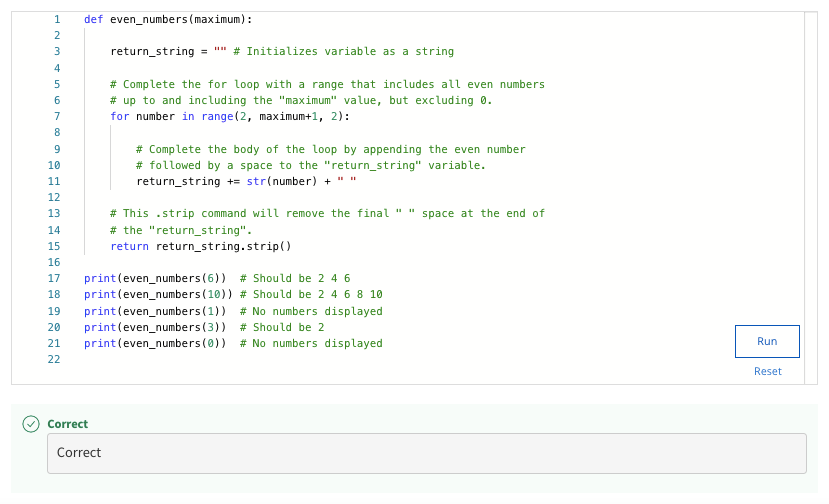#### 29. What happens when the Python interpreter executes a loop where a variable used inside the loop is not initialized?

• Nothing will happen
• Will produce a NameError stating the variable is not defined
• The variable will be auto-assigned a default value of 0
• Will produce a TypeError

#### 31. Fill in the blanks to print the even numbers from 2 to 12.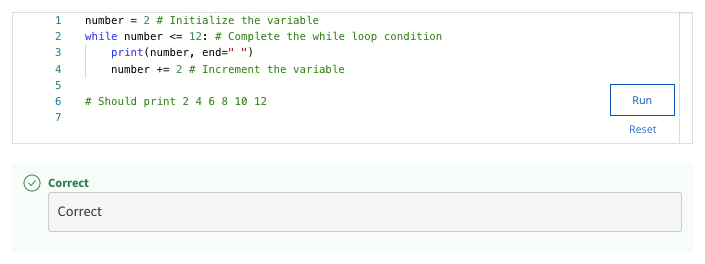#### 32. Find and correct the error in the for loop below. The loop should check each number from 1 to 5 and identify if the number is odd or even.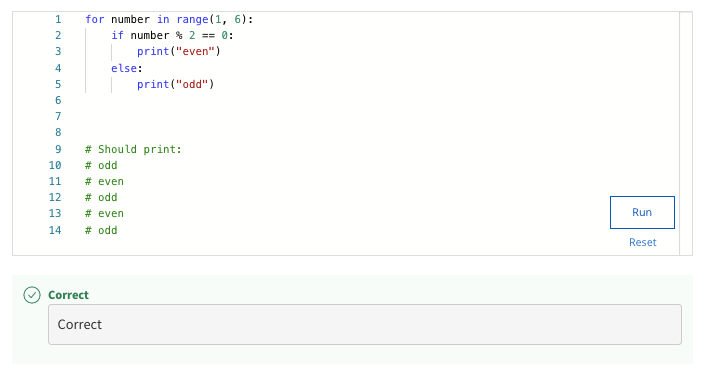#### 33. The following code is supposed to add together all numbers from x to 10. The code is returning an incorrect answer, what is the reason for this?x = 1sum = 5while x <= 10: sum += x x += 1print(sum)# Should print 55

• Should use a for loop instead of a while loop
• The code is not inside of a function
• Not incrementing the iterator (x)
• The “sum” variable is initialized with the wrong value

#### 34. The following code causes an infinite loop. Can you figure out what is incorrect?def test_code(num): x = num while x % 2 == 0: x = x / 2test_code(0)

• When called with 0, it triggers an infinite loop
• The modulo operator is used incorrectly
• Missing an else statement
• Missing the continue keyword

#### 35. Find and correct the error in the for loop below. The loop should print every even number from 2 to 12.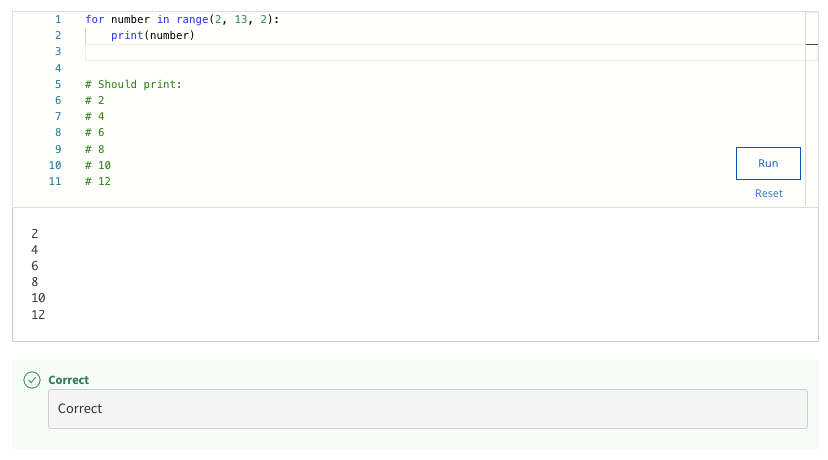#### 36. Fill in the blanks to complete the “all_numbers” function. This function should return a space-separated string of all numbers, from the starting “minimum” variable up to and including the “maximum” variable that's passed into the function. Complete the for loop so that a function call like “all_numbers(3,6)” will return the numbers “3 4 5 6”.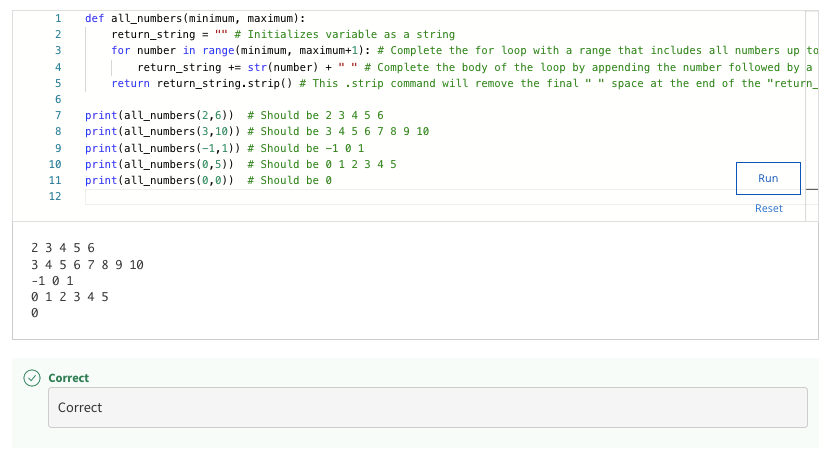#### 37. Fill in the blanks to complete the function “even_numbers(n)”. This function should count how many even numbers exist in a sequence from 0 to the given “n”number, where 0 counts as an even number. For example, even_numbers(25) should return 13, and even_numbers(6) should return 4.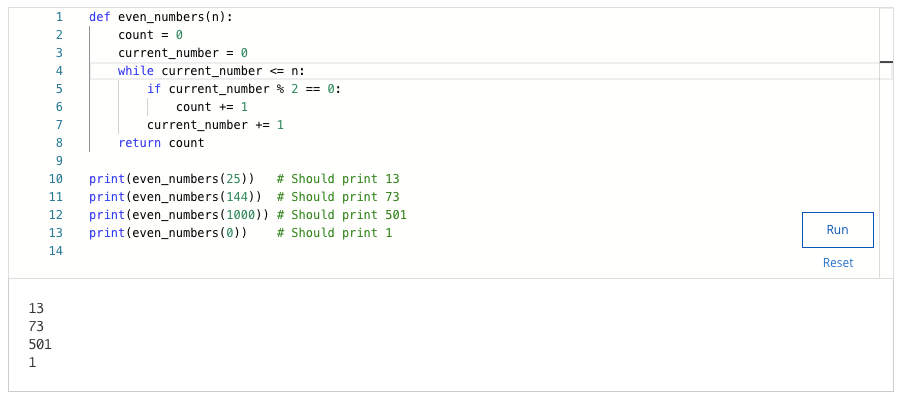## Shuffle Q/A 2

#### 38. Fill in the blanks to complete the “divisible” function. This function should count the number of values from 0 to the “max” parameter that are evenly divisible (no remainder) by the “divisor” parameter. Complete the code so that a function call like “divisible(100,10)” will return the number “10”.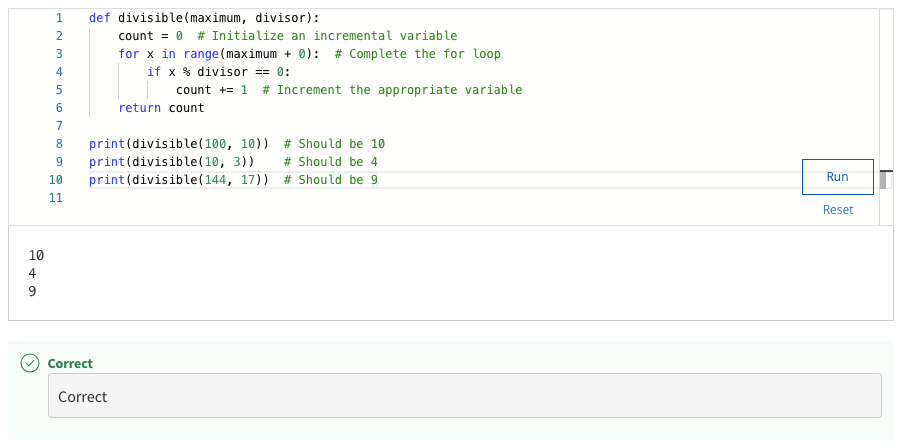#### 39. Fill in the blanks to complete the “rows_asterisks” function. This function should print rows of asterisks (*), where the number of rows is equal to the “rows” variable. The number of asterisks per row should correspond to the row number (row 1 should have 1 asterisk, row 2 should have 2 asterisks, etc.). Complete the code so that “row_asterisks(5)” will print:** ** * ** * * ** * * * *